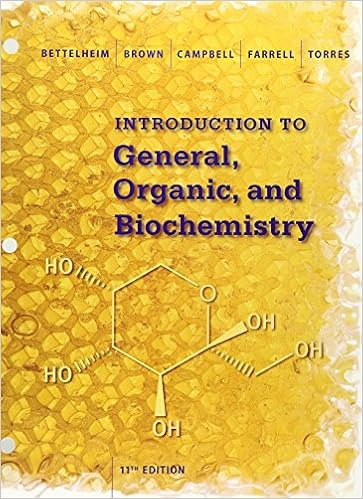# Calculate the total pressure a 120 atm b 150 atm c

• Test Prep
• 4
• 100% (5) 5 out of 5 people found this document helpful

This preview shows page 2 - 4 out of 4 pages.

##### We have textbook solutions for you!
The document you are viewing contains questions related to this textbook.The document you are viewing contains questions related to this textbook.
Chapter 8 / Exercise 8-116
Introduction to General, Organic and Biochemistry
BrownExpert Verified
89. Calculate the total pressure. A) 1.20 atm B) 1.50 atm C) 1.88 atm D) 2.01 atm E) none of these ANS: C
PTS: 1 DIF: Easy REF: 5.5 KEY: Chemistry | general chemistry | phases | gas | gas mixtures | Dalton's law of partial pressures MSC: Conceptual90. Calculate the ratio of PHe: PAr.
PTS: 1 DIF: Moderate REF: 5.5 KEY: Chemistry | general chemistry | phases | gas | gas mixtures | Dalton's law of partial pressures MSC: Conceptual91. The valve between a 5-L tank containing a gas at 9 atm and a 10-L tank containing a gas at 6 atm is opened. Calculate the final pressure in the tanks.
PTS: 1 DIF: Easy REF: 5.5 KEY: Chemistry | general chemistry | phases | gas | gas mixtures | Dalton's law of partial pressures MSC: Quantitative
##### We have textbook solutions for you!
The document you are viewing contains questions related to this textbook.The document you are viewing contains questions related to this textbook.
Chapter 8 / Exercise 8-116
Introduction to General, Organic and Biochemistry
BrownExpert Verified
92. A balloon contains an anesthetic mixture of cyclopropane (cp) and oxygen (O2) at 171 torr and 570. torr, respectively. What is the ratio of the number of moles of cyclopropane to moles of oxygen?
PTS: 1 DIF: Moderate REF: 5.2 KEY: Chemistry | general chemistry | phases | gas | empirical gas laws | Avogadro's law MSC: Quantitative
•••